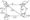# VTU Previous Year Question Papers Logic design January 2008

1. Answer any FIVE full questions.

2. Assume missing data if any suitably.

1 a. Two motors M2 and Mj are controlled by three sensors 83, S2 and Si. One motor M2 is to run any time all three sensors are on. The other motor is to run whenever sensors S2 or Si but not both are on and S3 is off. For all sensor combinations where Mi is on, M2 is to be off except when all the three sensors are off and then both motors must remain off. Construct the truth table and write the Boolean output equation.

b. Simplify using Karnaugh map. Write the Boolean equation and realize using NAND

gates.D = f (w, x, y, z) = £(0,2,4,6,8)+Ed(l 0,11,1 2,13,14,15).

c. Simplify P = f(a,b,c,) = 2(0,1,4,5,7)using two variable Karnaugh map. Write the Boolean equation and realize using logic gates.

2 a. Simplify using Karnaugh map L = f(a,b,c,d) = tt(2,3,4,6,7,1 0,11,12)

b.    Simplify using Quine Me Cluskey tabulation algorithm-

V = f(a,b,c,d) = £(2,3,4,5,13,15)+ Σ d(8,9,10,11)

3 a. Design a combinational circuit that will multiply two two-bit binary values.

b. Design a 4 to 16 decoder using two 3 to 8 decoder (74LS138).

c. Design a keypad interface to a digital system using ten line BCD encoder (74LS147).

4 a. Design a binary full subtractor using minimum number of gates.

b. Explain the terms

i)      Ripple – carry propagation

ii)     Propagation delay

iv)    Iterative design.

c. Realize F = f(x,y,z,) = 2(l,2,4,5,7) using 8 -to – 1 multiplexer (74LS151), Design a two bit binary magnitude comparator.

d. Explain with timing diagram the working of a S. R latch as a switch debouncer.

5 a.Explain the working of a Master – slave JK Flip-Flop with functional table and timing diagram. Show how race around condition of master-slave SR Flip-Flop is over come.

b. What is the significance of edge triggering? Explain the working of edge triggered D-flip-flop and T-flip-flop with their functional table.

6 a. Obtain the characteristic equation for a SR flip-flop

b. With a neat circuit diagram, explain the working of a universal shift register. 3. Design a synchronous Mod-6 counter using clocked J K flip-flop.

7 a. Explain mealy and Moore sequential circuit models.

b.    For the state machine Mj shown in Fig. Q 7(b), obtain

i) State table

ii) Transition table                ”

iii) Exaltation table for T flip-flop

iv) Logic circuit for T exaltation realization.

8 a. Construct a mealy state diagram that will detect a serial sequence of 10110. When the input pattern has bee detected, cause an output Z to be asserted high.

b. Design a cyclic modulo-8 synchronous counter using J-K flip-flop that will count the number of occurrences of an input; that is, the number of times it is a 1. The input variable X must be coincident with the clock to be counted. The counter is to count in binary.